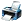# p(x) is a polynomial of degree 5 and has extremum at -1 and 1 and lim x -0(p(x)/x cube -2)=4

4,573 views

p(x) is a polynomial of degree 5 and has extremum at -1 and 1 and lim x -0(p(x)/x^3 -2)=4 if m and M are maximum and minimum value of y=f'(x) on the set A={x|x^2+6x<5x} then m/M isposted Oct 27, 2016

consider p(x)=ax^5+bx^4+cx^3+dx^2+ex+f
Now d=e=f=0 as lim x -0(p(x)/x^3 -2)=4 so power of x<3 causing the destabilisation of finite value on right side.
so our polynomial p(x) becomes p(x)=ax^5+bx^4+cx^3
Now, putting all values in limit and we get c-2=4 implies c=6.
Also p(x) has extremum at -1 and 1 so putting the value firstly x=-1 and equating dp(x)/dx=0.
We get equation (1)
Using same for x=1 and we get equation (2)
Solving equation (1) and (2) we get "a" and "b"
Putting value of "a", "b", "c" in p(x) we get our desired polynomial.answer Oct 27, 2016
I didn't get about second part of this question as i don't know these two questions are same or not as these two seems to be different.
Where does y=f'x come from ? Is your set properly defined ? You wrote A={x|x^2+6x<5x} . Is it correct ? Then it simply becomes A={x|x^2+x<0} . Please make desired correction .answer Oct 28, 2016